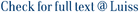Indexing highly repetitive texts—such as genomic databases, software repositories and versioned text collections—has become an important problem since the turn of the millennium. A relevant compressibility measure for repetitive texts is r, the number of runs in their Burrows-Wheeler Transforms (BWTs). One of the earliest indexes for repetitive collections, the Run-Length FM-index, used O(r) space and was able to efficiently count the number of occurrences of a pattern of length m in a text of length n (in O(m log log n) time, with current techniques). However, it was unable to locate the positions of those occurrences efficiently within a space bounded in terms of r. In this article, we close this long-standing problem, showing how to extend the Run-Length FM-index so that it can locate the occ occurrences efficiently (in O(occ log log n) time) within O(r) space. By raising the space to O(r log log n), our index counts the occurrences in optimal time, O(m), and locates them in optimal time as well, O(m + occ). By further raising the space by an O(w/ log σ) factor, where σ is the alphabet size and w = Ω (log n) is the RAM machine size in bits, we support count and locate in O(⌈ m log (σ)/w ⌉) and O(⌈ m log (σ)/w ⌉ + occ) time, which is optimal in the packed setting and had not been obtained before in compressed space. We also describe a structure using O(r log (n/r)) space that replaces the text and extracts any text substring of length ℓ in the almost-optimal time O(log (n/r)+ℓ log (σ)/w). Within that space, we similarly provide access to arbitrary suffix array, inverse suffix array, and longest common prefix array cells in time O(log (n/r)), and extend these capabilities to full suffix tree functionality, typically in O(log (n/r)) time per operation. Our experiments show that our O(r)-space index outperforms the space-competitive alternatives by 1--2 orders of magnitude in time. Competitive implementations of the original FM-index are outperformed by 1--2 orders of magnitude in space and/or 2--3 in time.

Fully Functional Suffix Trees and Optimal Text Searching in BWT-Runs Bounded Space / Gagie, Travis; Navarro, Gonzalo; Prezza, Nicola. - In: JOURNAL OF THE ASSOCIATION FOR COMPUTING MACHINERY. - ISSN 0004-5411. - 67:1(2020), pp. 1-54. [10.1145/3375890]

### Fully Functional Suffix Trees and Optimal Text Searching in BWT-Runs Bounded Space

#### Abstract

Indexing highly repetitive texts—such as genomic databases, software repositories and versioned text collections—has become an important problem since the turn of the millennium. A relevant compressibility measure for repetitive texts is r, the number of runs in their Burrows-Wheeler Transforms (BWTs). One of the earliest indexes for repetitive collections, the Run-Length FM-index, used O(r) space and was able to efficiently count the number of occurrences of a pattern of length m in a text of length n (in O(m log log n) time, with current techniques). However, it was unable to locate the positions of those occurrences efficiently within a space bounded in terms of r. In this article, we close this long-standing problem, showing how to extend the Run-Length FM-index so that it can locate the occ occurrences efficiently (in O(occ log log n) time) within O(r) space. By raising the space to O(r log log n), our index counts the occurrences in optimal time, O(m), and locates them in optimal time as well, O(m + occ). By further raising the space by an O(w/ log σ) factor, where σ is the alphabet size and w = Ω (log n) is the RAM machine size in bits, we support count and locate in O(⌈ m log (σ)/w ⌉) and O(⌈ m log (σ)/w ⌉ + occ) time, which is optimal in the packed setting and had not been obtained before in compressed space. We also describe a structure using O(r log (n/r)) space that replaces the text and extracts any text substring of length ℓ in the almost-optimal time O(log (n/r)+ℓ log (σ)/w). Within that space, we similarly provide access to arbitrary suffix array, inverse suffix array, and longest common prefix array cells in time O(log (n/r)), and extend these capabilities to full suffix tree functionality, typically in O(log (n/r)) time per operation. Our experiments show that our O(r)-space index outperforms the space-competitive alternatives by 1--2 orders of magnitude in time. Competitive implementations of the original FM-index are outperformed by 1--2 orders of magnitude in space and/or 2--3 in time.
##### Scheda breve Scheda completa Scheda completa (DC)2020
Theory of computation, Design and analysis of algorithms, Data structures design and analysis, Pattern matching
Fully Functional Suffix Trees and Optimal Text Searching in BWT-Runs Bounded Space / Gagie, Travis; Navarro, Gonzalo; Prezza, Nicola. - In: JOURNAL OF THE ASSOCIATION FOR COMPUTING MACHINERY. - ISSN 0004-5411. - 67:1(2020), pp. 1-54. [10.1145/3375890]
File in questo prodotto:
File
jacm.pdf

Open Access

Descrizione: articolo principale
Tipologia: Documento in Pre-print
Licenza: DRM (Digital rights management) non definiti
Dimensione 637.98 kB
Utilizza questo identificativo per citare o creare un link a questo documento: `https://hdl.handle.net/11385/192324`
•79
•40